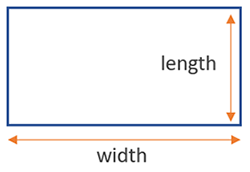# Area of a Rectangle Calculator

Use this calculator to easily calculate the area of a rectangle given its sides (length and width) in any metric: mm, cm, meters, km, inches, feet, yards, miles...

### Calculation results

12 cm2 Rectangle area
Share calculator:

Embed this tool:
get code

## Area of a rectangle formula

The formula for the area of a rectangle is width x height, as seen in the figure below:All you need are two measurements and you can calculate its perimeter by hand, or by using our perimeter of a rectangle calculator above. The result will be in the unit the width and height are measured in, but squared, e.g. mm2, cm2, m2, km2 or in2, ft2, yd2, mi2.

## How to calculate the area of a rectangle?

The calculation is straightforward using the formula above: just make sure you have the measurement of the width and height in the same units, and then multiply them. Don't forget to express the result in the squared unit, and you are good to go.

## Example: find the area of a rectangle

The area of any rectangular place is or surface is its length multiplied by its width. For example, a garden shaped as a rectangle with a length of 10 yards and width of 3 yards has an area of 10 x 3 = 30 square yards. A rectangular bedroom with one wall being 15 feet long and the other being 12 feet long is simply 12 x 15 = 180 square feet.

Since in multiplication the order in which the numbers are multiplied does not matter, you need not worry about switching the places of the two measurements.

## Practical applications

Area of a rectangle calculations have a vast array of practical applications: construction, landscaping, internal decoration, architecture, engineering, physics, and so on and so forth. Irregularly shaped areas are often divided into several rectangles when one needs to calculate their area, but can't do a precise calculation. This is how the human body surface area was calculated as well: subject's bodies were covered in stripes of paraffin, which were then removed, sliced and measured.

#### Cite this calculator & page

If you'd like to cite this online calculator resource and information as provided on the page, you can use the following citation:
Georgiev G.Z., "Area of a Rectangle Calculator", [online] Available at: https://www.gigacalculator.com/calculators/area-of-rectangle-calculator.php URL [Accessed Date: 27 Mar, 2023].